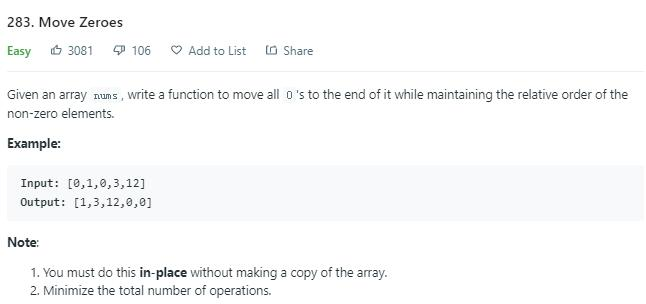# 题目描述（简单难度）# 解法一

i 指针遇到非零元素就和 j 指针指向的元素交换，j 指针然后后移。

0,1,0,3,12 为例模拟一下过程。

0,1,0,3,12
^
i
j

nums[i] == 0, i 后移
0,1,0,3,12
^ ^
j i

nums[i] != 0, 交换和 j 指向的元素, i 后移, j 后移
1,0,0,3,12
^ ^
j i

nums[i] == 0, i 后移
1,0,0,3,12
^   ^
j   i

nums[i] != 0, 交换和 j 指向的元素, i 后移, j 后移
1,3,0,0,12
^   ^
j   i

nums[i] != 0, 交换和 j 指向的元素, i 后移, j 后移, 遍历结束
1,3,12,0,0
^   ^
j   i


public void moveZeroes(int[] nums) {
int j = 0;
for (int i = 0; i < nums.length; i++) {
//不等于 0 就交换
if (nums[i] != 0) {
int temp = nums[j];
nums[j] = nums[i];
nums[i] = temp;
j++;
}
}
}